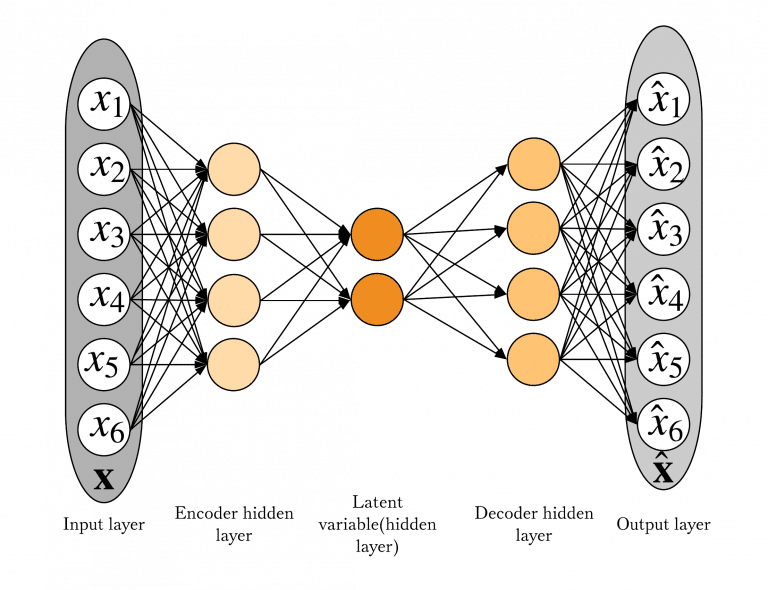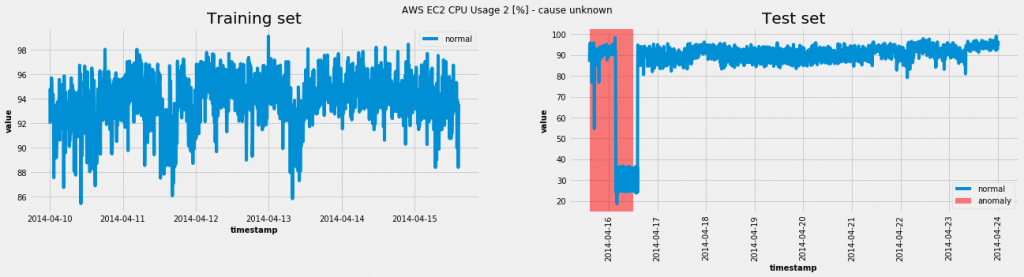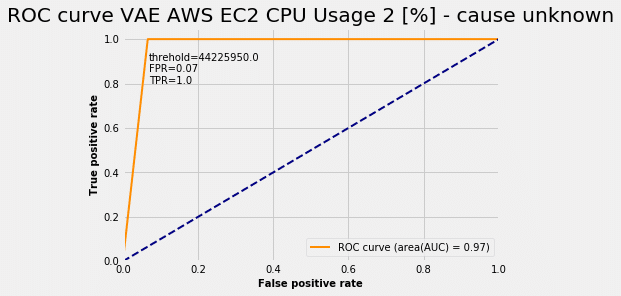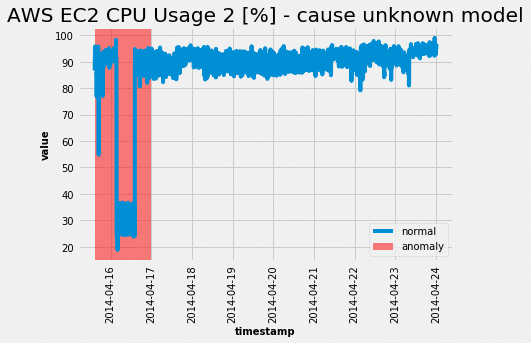# How to perform Time Series Clustering using ML

Time series is a term that you must or would have faced in your Data Science career. If you are completely new to this, don’t worry, it is really intuitive. What is actually a Time Series? It is a collection of data points collected at constant time intervals. So, we have a history data about some feature, which was collected every day or at some other time interval. Important thing is that it has to be the same interval every time. In the next picture you can see one example of Time Series.Within the Time Series, we could have different applications. There are two ways that we could use Time Series: we could use just historical data and analyse it, which is called Time Series analysis, or we could use historical data in order to predict future values of it, which is called Time Series Forecasting. In some later blogs, you will find a little more about Forecasting, but now, we are focusing on analysis.

# Time Series Analysis

Time series analysis is a popular field of Data Science which includes developing models (statistical or machine learning models) that can describe observed Time Series in the best way and maybe explain underlying causes and patterns. In order to do so, we can use several techniques, like anomaly detection, create autoregressive models, recognize trend patterns, measure similarity of Time Series… We can already see that it could be useful in many different industries. We found it useful in a few of our projects, when we wanted to find answers for questions like:

• Why does the data change this way?
• Are there any patterns in behaviour of some time series features?
• Which time series show similar patterns?
• etc.

We have done detailed research in order to find the best way for answering those questions. Finally, we have decided to try Time Series clustering.

## Time Series clustering

Main goal of Time Series clustering is to partition Time Series data into groups based on similarity or distance, so that Time Series in the same cluster are similar. At first, it looks like that it is the same problem as any basic clustering, but here we have specific data and specific decisions to make before fitting the model.Image source: Comparing Time-Series Clustering Algorithms in R Using the dtwclust Package by Alexis Sarda-Espinosa

The main decisions that we need to make are:

• how to measure the similarity between Time Series
• how to compress the Time Series data or reduce dimension and
• what algorithm to use for clustering

## Similarity measures

There are many ways to define similarity measures for clustering. First choice for a similarity measure would be Euclidean distance. The problem with using the Euclidean distance measure is that it often produces pessimistic similarity measures when it encounters distortion in the time axis. Also, we could not compare Time Series that are not the same length. The way to deal with this is to use Dynamic Time Warping as a similarity measure.Dynamic time warping finds the optimal non-linear alignment between two Time Series. This similarity measure requires a blog for itself. If you are interested more in how this works, you can find an excellent explanation in this video. But why is this useful? If we want to detect the same pattern in some data, maybe we don’t care for the exact time that this pattern happened, but we just want to detect it and put them in the same cluster. For example, if we are analysing retailer’s sales for many stores, we want to detect where we have sudden peak in sales, cluster them and then analyze further to see what have caused that, no matter when that peak happened in time.Image source: Dynamic time warping under pointwise shape context by Zheng Zhang, PingTang and Rubing Duan

## Algorithms for clustering Time Series

The other important step is to decide which clustering algorithm to use. Here, I have listed those which enable using DTW as a similarity measure, their characteristics and requirements.In our case, KMeans turned out to be the best algorithm for Time Series clustering. It was the fastest and the simplest solution. Nevertheless, we encourage you to try out all of them, because results depend on the data that you have been using.

## Evaluation metrics

Of course, it is not enough to just cluster your data. You have another problem – to find the optimal number of clusters. In order to do that, you have to define an evaluation metric which you will optimize. Here are the most widely used evaluation metrics for clustering.After research, it was concluded that we can rely on the Silhouette score, but we will also monitor Calinski-Harabasz index (because Davies-Bouldin is restricted to using Euclidean distance for distance metric, which we are not using). It is a good practice to have two evaluation metrics, just to be sure that gained results are optimal.

# Final notes

The most important thing when doing Time Series clustering is to understand data and domain that data comes from. Maybe our evaluation metric gives us one number for optimal clusters, but we should make the final decision about it when we analyze results and see how we can interpret the results. If you are working on this with some domain expert, then you are lucky – he can help you to find the best solution in the most efficient way. 🙂

Cover photo credits: https://unsplash.com/photos/nN5L5GXKFz8

# Time series Anomaly Detection using a Variational Autoencoder (VAE)

## Why time series anomaly detection?

Let’s say you are tracking a large number of business-related or technical KPIs (that may have seasonality and noise). It is in your interest to automatically isolate a time window for a single KPI whose behavior deviates from normal behavior (contextual anomaly – for the definition refer to this post). When you have the problematic time window at hand you can further explore the values of that KPI. You can then link the anomaly to an event which caused the unexpected behavior. Most importantly, you can then act on the information.

To do the automatic time window isolation we need a time series anomaly detection machine learning model. The goal of this post is to introduce a probabilistic neural network (VAE) as a time series machine learning model and explore its use in the area of anomaly detection. As this post tries to reduce the math as much as possible, it does require some neural network and probability knowledge.

## Background

As Valentina mentioned in her post there are three different approaches to anomaly detection using machine learning based on the availability of labels:

1. unsupervised anomaly detection
2. semi-supervised anomaly detection
3. supervised anomaly detection

Someone who has knowledge of the domain needs to assign labels manually. Therefore, acquiring precise and extensive labels is a time consuming and an expensive process. I’ve deliberately put unsupervised as the first approach, since it doesn’t require labels. It does, however, require that normal instances outnumber the abnormal ones. Not only do we require an unsupervised model, we also require it to be good at modeling non-linearities.

## What model? Enter neural networks…Historically, different kinds of neural networks have had success with modeling complex non-linear data (e.g. image, sound and text data). However, universal function approximators that they are, they have inevitably found their way into modeling tabular data. One interesting type of tabular data modeling is time-series modeling.

A model that has made the transition from complex data to tabular data is an Autoencoder(AE). Autoencoder consists of two parts – encoder and decoder. It tries to learn a smaller representation of its input (encoder) and then reconstruct its input from that smaller representation (decoder). An anomaly score is designed to correspond to the reconstruction error.

Autoencoder has a probabilistic sibling Variational Autoencoder(VAE), a Bayesian neural network. It tries not to reconstruct the original input, but the (chosen) distribution’s parameters of the output. An anomaly score is designed to correspond to an – anomaly probability. Choosing a distribution is a problem-dependent task and it can also be a research path. Now we delve into slightly more technical details.

Both AE and VAE use a sliding window of KPI values as an input. Model performance is mainly determined by the size of the sliding window.

##The smaller representation in the VAE context is called a latent variable and it has a prior distribution (chosen to be the Normal distribution). The encoder is its posterior distribution and the decoder is its likelihood distribution. Both of them are Normal distribution in our problem. A forward pass would be:

1. Encode an instance into a mean value and standard deviation of latent variable
2. Sample from the latent variable’s distribution
3. Decode the sample into a mean value and standard deviation of the output variable
4. Sample from the output variable’s distribution

Variational Autoencoder as probabilistic neural network (also named a Bayesian neural network). It is also a type of a graphical model. An in-depth description of graphical models can be found in Chapter 8 of Christopher Bishop‘s Machine Learning and Pattern Recongnition.

A TensorFlow definition of the model:

```class VAE(object):
def __init__(self, kpi, z_dim=None, n_dim=None, hidden_layer_sz=None):
"""
Args:
z_dim : dimension of latent space.
n_dim : dimension of input data.
"""
if not z_dim or not n_dim:
raise ValueError("You should set z_dim"
"(latent space) dimension and your input n_dim."
" \n            ")

tf.reset_default_graph()

def make_prior(code_size):
loc = tf.zeros(code_size)
scale = tf.ones(code_size)
return tfd.MultivariateNormalDiag(loc, scale)

self.z_dim = z_dim
self.n_dim = n_dim
self.kpi = kpi
self.dense_size = hidden_layer_sz

self.input = tf.placeholder(dtype=tf.float32,shape=[None, n_dim], name='KPI_data')
self.batch_size = tf.placeholder(tf.int64, name="init_batch_size")

# tf.data api
dataset = tf.data.Dataset.from_tensor_slices(self.input).repeat() \
.batch(self.batch_size)
self.ite = dataset.make_initializable_iterator()
self.x = self.ite.get_next()

# Define the model.
self.prior = make_prior(code_size=self.z_dim)
x = tf.contrib.layers.flatten(self.x)
x = tf.layers.dense(x, self.dense_size, tf.nn.relu)
x = tf.layers.dense(x, self.dense_size, tf.nn.relu)
loc = tf.layers.dense(x, self.z_dim)
scale = tf.layers.dense(x, self.z_dim , tf.nn.softplus)
self.posterior = tfd.MultivariateNormalDiag(loc, scale)
self.code = self.posterior.sample()

# Define the loss.
x = self.code
x = tf.layers.dense(x, self.dense_size, tf.nn.relu)
x = tf.layers.dense(x, self.dense_size, tf.nn.relu)
loc = tf.layers.dense(x, self.n_dim)
scale = tf.layers.dense(x, self.n_dim , tf.nn.softplus)
self.decoder = tfd.MultivariateNormalDiag(loc, scale)
self.likelihood = self.decoder.log_prob(self.x)
self.divergence = tf.contrib.distributions.kl_divergence(self.posterior, self.prior)
self.elbo = tf.reduce_mean(self.likelihood - self.divergence)
self._cost = -self.elbo

self.saver = tf.train.Saver()
self.sess = tf.Session()

```
```def fit(self, Xs, learning_rate=0.001, num_epochs=10, batch_sz=200, verbose=True):

batches_per_epoch = int(np.ceil(len(Xs) / batch_sz))
print("\n")
print("Training anomaly detector/dimensionalty reduction VAE for KPI",self.kpi)
print("\n")
print("There are",batches_per_epoch, "batches per epoch")
start = timer()

self.sess.run(tf.global_variables_initializer())

for epoch in range(num_epochs):
train_error = 0

self.sess.run(
self.ite.initializer,
feed_dict={
self.input: Xs,
self.batch_size: batch_sz})

for step in range(batches_per_epoch):
_, loss = self.sess.run([self.optimize, self._cost])
train_error += loss
if step == (batches_per_epoch - 1):
mean_loss = train_error / batches_per_epoch
if verbose:
print(
"Epoch {:^6} Loss {:0.5f}"  .format(
epoch + 1, mean_loss))

if train_error == np.nan:
return False
end = timer()
print("\n")
print("Training time {:0.2f} minutes".format((end - start) / (60)))
return True```

## Theory is great… What about real world?

Using the model on one of the data sets from the Numenta Anomaly Benchmark(NAB):In this case the model was able to achieve a true positive rate (TPR = 1.0) and a false positive rate (FPR = 0.07). For various anomaly probability thresholds we get a ROC curve:Choosing the threshold read from the ROC curve plot we get the following from the test set:Just as the ROC curve suggested, the model was able to completely capture the abnormal behavior. Alas, as all neural network models are in need of hyperparameter tuning, this beast is no exception. However the only hyperparameter that can greatly affect the performance is the size of the sliding window.

I hope I was successful in introducing this fairly complex model in simple terms. I encourage you to try the model on other data sets available from here.

Keep on learning and things solving!

# Forecasting with ARIMA

One of the most challenging machine learning problems is predicting some output based on the history of its previous values. The complexity of the problem multiplies as new features and constraints are added to analysis. Thus, in time series analysis it is not always enough to use previous values only, there often are many features that could impact on the output which should be predicted. There are numerous models and algorithms developed for this kind of problem. In this article, and series of articles that follows, several models, such as ARIMA, VAR and  Facebook Prophet, will be introduced and finally compared to see which one is best suitable for different kinds of problems.

ARIMA stands for autoregressive integrated moving average model, which produces forecasts based upon prior values in the time series (AR terms) and the errors made by previous predictions (MA terms), with possibility to work with non-stationary data, since it allows us to initially differentiate data to eliminate the non-stationarity (I term).

PyFlux library offers a very good implementation of ARIMA model in Python. It has useful documentation, followed by examples and it is very easy to use. To run  this model, we need to determine the optimal value for each of the parameters this function takes – AR, MA and I terms.

#### Problem definition

Data used for analysis include 125 instances, historical values, gathered during time period January-May 2017. Data take values mostly between 20-40, with deviation of +-15, which depends on the object that is observed. The problem is to predict the output for a given interval, based on these historical data. This is how our data look like.Three things that should be investigated before running a model are time series stationarity, trend and seasonality, since each of them could negatively affect results obtained. That will tell us if we should differentiate data before training.

A rough conclusion that data is not stationary could be made by simply looking at the plot, but there is a little more elegant solution, which allows us to exactly determine if data is stationary – a Dickey-Fuller test, which has its implementation in StatsModels library. Here’s one example of how it can be used, taken from here.

These are results of a DF test applied to our data.The test statistics is higher than the critical value, which leads to a conclusion that we cannot accept the null hypothesis of data stationarity.

The most popular technique used to make data stationary and eliminate trend and seasonality is differentiating.

ARIMA function in PyFlux library has a parameter that is used to set number of differencing order. In most cases, first order of differencing will do the job, but sometimes it is needed to differentiate data several times.

There are enough guidelines on how to determine the order of differencing and there is an excellent post that summarizes rules for identifying a proper ARIMA model, and set its parameters (https://people.duke.edu/~rnau/arimrule.htm ).

So, our data now look like this.After re-running a stationarity test on differentiated data, we can see the following results.A test statistics is now less than the critical value of 5% confidence interval, and we can adopt the null hypothesis that data is stationary.

Looks decent, so we could continue with analysis and run a model.

#### Set up parameters

The trickiest part of running an ARIMA model is determining the optimal number for AR and MA terms. There are two additional functions that can be used to solve this problem: ACF (autocorrelation function) and PACF (partial autocorrelation function). Their implementation can also be found in StatsModels library. Basically, both are used to determine how past and future data points are related in a time series, where the ACF function measures the correlation between two successive points, and the PACF tries to remove the transitive dependency between points (if a depends on b, and b depends on c, than a depends on c.)

Autocorrelation function can be used to determine the optimal number for MA terms by the following rule: the lag value where the ACF chart crosses the upper confidence interval for the first time is the recommended value for MA parameter. In this case, it is 29.Partial autocorrelation function is used to determine the optimal value for AR parameter in ARIMA function (the same rule as previous is used).The optimal value for AR parameter is 2.

Since we have optimal parameter values, we are able to run a model. One additional parameter that could be set is a distribution family of the time series – by default it is Normal but other options include Student-t, Laplace, Cauchy, etc.

#### Running a model

model = ARIMA(data=working_data, ar=2, ma=29, integ=1, target=’output’, family=pf.Normal())

The model is fitted with MLE – maximum likelihood point mass estimate method, which is used to estimate model parameters, by finding particular parametric values that make the observed results the most probable given the model.

Here’s the summary.AIC and BIC are used as model performance measures. AIC is acronym for Akaike Information Criteria and it is widely used as a measure of a statistical model. It basically quantifies the goodness of fit, and the simplicity of the model into a single statistics. BIC is a Bayesian information criteria. If a model is estimated on a training set, BIC score gives an estimate of the model performance on a testing set. The main rule is “the lower, the better”, and those two can be mixed to choose the best-fit model among several others.

And this is what results look like graphically.Pretty powerful, right?

Well, at least we thought so, until we came up to something like this.We were looking for a more stable, accurate model and weren’t satisfied with ARIMA and its 3.56 RMSE, so we dug deeper and found out that the output we’re trying to predict depends on many other features that should also be included in modeling. That’s when we applied a VAR model that allows us to introduce exogenous variables and train an autoregressive model which takes into account values of these external features beside the historical values of the output that should be predicted. We will be talking about it more in the next post.